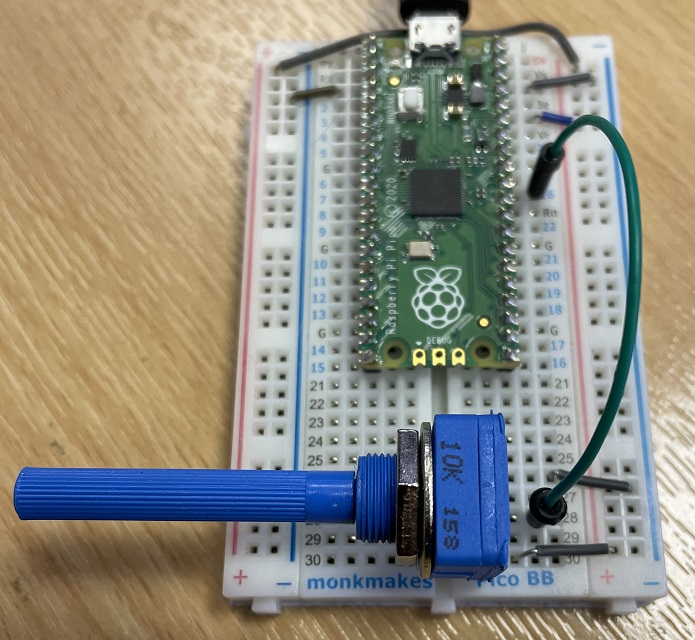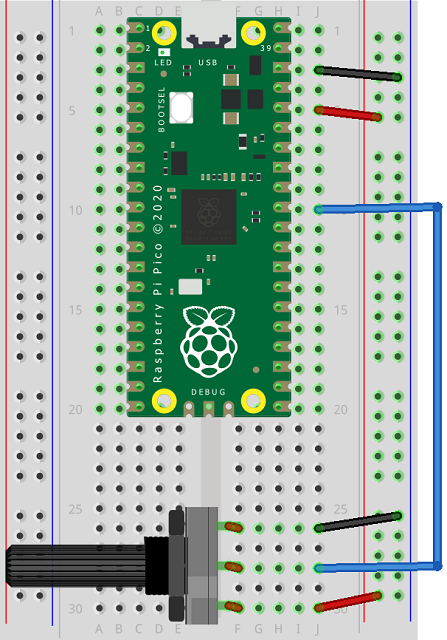A potentiometer is a simple way to check how to read analog components. I have connected this one 3V3, GND and the middle pin to GP26.Here is the Fritzing diagram showing that,In the first program, I just get and print a simple reading from the ADC.

```import board
from time import sleep

while True:
print(pot.value)
sleep(1)
```

This program converts that to a voltage level.

```import board
from time import sleep

voltage_converter = 3.3 / 65535

while True:
volts = round(pot.value * voltage_converter,1)
print(str(volts) + "V")
sleep(1)
```

In this final program, I just want to have a byte representing the reading.

```import board
from time import sleep